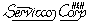Sampling Plans Site Map
Applications Area Topics:

AQL Primer
Audit for Bed Bugs
Inventory Sampling
Xbar Charts
Color Measurement
Reliability Growth Test Plan
Wearout and MTBF
TP414 -vs- Mil-Std-414
Switching Rules

 Xbar Charts and Sampling Plans visitors=Process control and product acceptance

As you develop toward more economical and more effective procedures for process control and for product acceptance, it pays to understand the relationship of Xbar charts to acceptance sampling plans.The strong point of the Xbar chart is to provide some information that the sampling plans can't -- process understanding is gained by analyzing the patterns over time. Also graphs communicate well. .The OC-Curve of a control chart will show why Shewhart charts are not suitable for product acceptance.

The relationship

Xbar control charts are equivalent to sampling plans for the mean carried out repeatedly, and the results plotted and analyzed in sequence. The control limits are exactly the same as the acceptance limits for a targeted (two-sided) sampling plan for the mean.

To be equivalent to a Shewhart chart, the sampling plan for the mean must have these risks:
alpha=0.0027 (two-sided) or alpha=0.00135(one-side) and
Beta=0.5.

How to calculate the sample size for arbitrarily chosen control limits:

You can use TP414 to calculate the sample size for Xbar charts, starting with the control limits as input.

Calculate the sample size required for selected control limits by entering these performance requirements into TP414 using: [Mean] [Both] [Performance]

0.00135, 0.5, LCL, Target, Target, UCL

Where LCL and UCL are chosen control limits, and Target is the center line.

How to calculate control limits for arbitrary chosen sample size:

You can use TP414 to calculate the control limits of an Xbar chart (starting with the sample size as input) by:
Use options [P] [L] and enter:  alpha=0.00135, Beta=0.5, 1,2

Now use the up-arrow to back up(alpha and beta defaults have been set to the proper values).
Use options [D] [L], and enter n,0

Calculate the control limits as: center line +- AQLML.

How to calculate the OC-Curve and ARL-Curve of an Xbar chart:

You can use TP414 to calculate the OC-Curve and ARL-Curve of an existing  Xbar chart by entering it into the program as follows:

Use options [M] [B] [D] and enter the values for n,LCL,UCL

View the OC-Curve and ARL-Curve table. The OC-Curve will show that the population mean can be outside of the control limits and still have a Pa=0.5 (50 percent probability of acceptance). For this reason, Shewhart charts are not suitable for product acceptance.